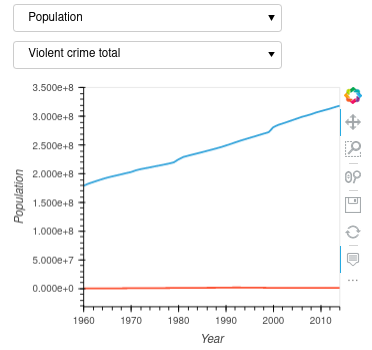# Is it possible to overlay plots created by the pipeline output from interactive()?

In the hvPlot tutorial home page, there is an example of overlaying two plots generated from dataframe.hvplot() directly. This is from the ability of HoloViews.

``````import hvplot.pandas  # noqa
from hvplot.sample_data import us_crime as df

plot1 = df.hvplot(x='Year', y='Violent Crime rate', width=400)
plot2 = df.hvplot(x='Year', y='Burglary rate', width=400)
plot1 + plot2
``````

However I haven’t seen any example of overlay of two plots turned from the data pipline by interactive(). Ideally, I would like to created two plots with their own individual widgets from the data pipeline and be able to overlay them with common x-axis and y-axis and eventually turn them into a dashboard app. It seems the " * " operator does not work with two interactive data pipelines.

If looked at more specifically, a dataframe.hvplot generates a type of `holoviews.core.overlay` object while dataframe.interactive() generates a `hvplot.interactive.Interactive` object.

Is it possible to overlay them with hvPlot, or should drill down to HoloViews? I guess the question is how to obtain the underlying holoviews figure object from `hvplot.interactive.Interactive`.

Yes.

``````import hvplot.pandas  # noqa
import panel as pn
from hvplot.sample_data import us_crime

pn.extension()

opts = list(df.columns)

w1 = pn.widgets.Select(value=opts, options=opts)
w2 = pn.widgets.Select(value=opts, options=opts)

p1 = df.interactive().hvplot(x="Year", y=w1, width=400)
p2 = df.interactive().hvplot(x="Year", y=w2, width=400)

p1 * p2
``````1 Like